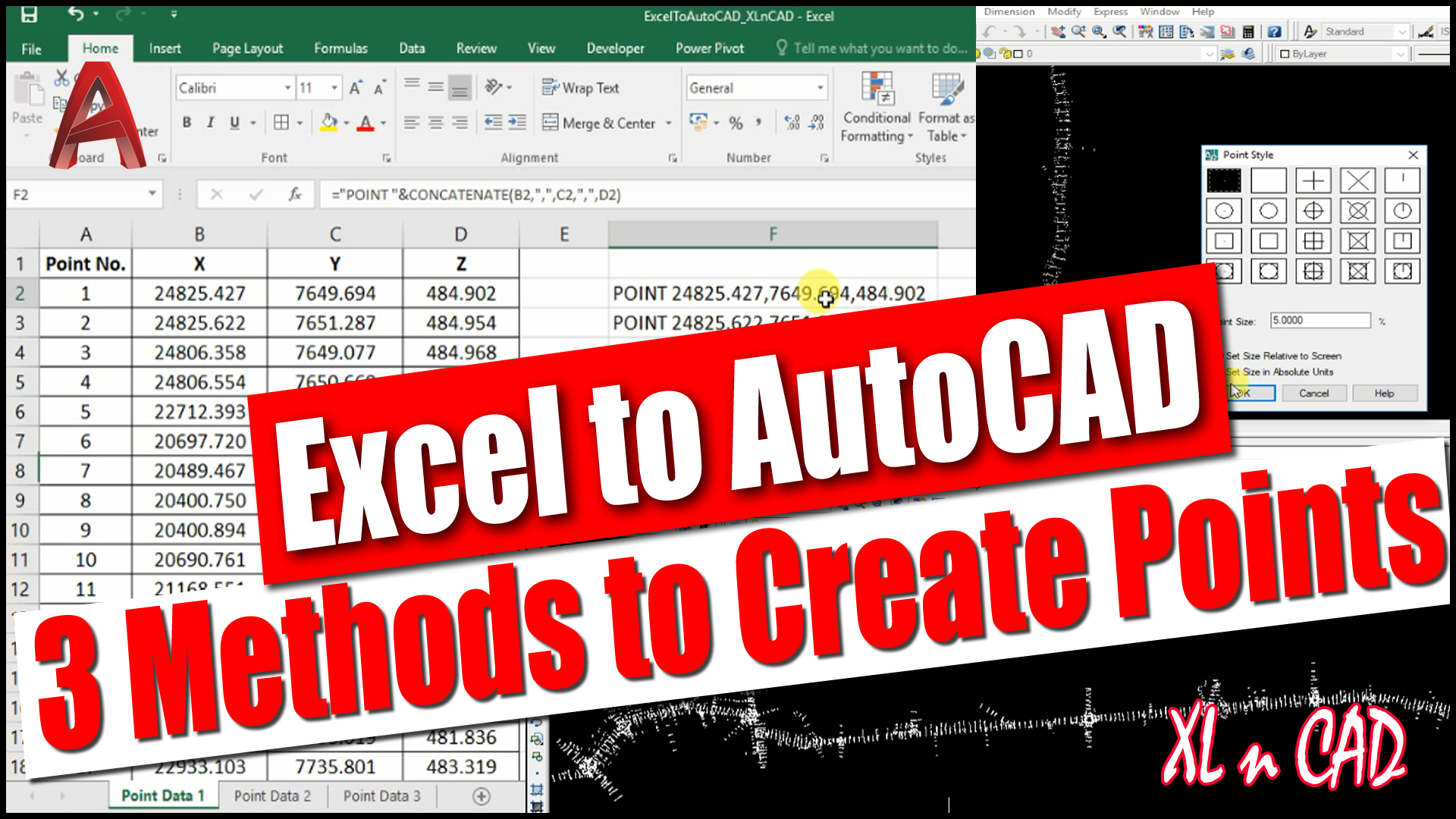# Import X, Y, Z Coordinates from Excel to AutoCADFollowing is a video explaining this technique and if you prefer video over reading, I recommend you to watch it.

As shown in this pic below, we have X, Y, Z coordinates, otherwise Easting, Northing & Reduced levels of more than 10000 points in an Excel sheet.

To create points using this data (to Export this data into an AutoCAD drawing), we have to convert this data into the language that AutoCAD can understand.

Type in POINT in the command bar and hit the Space bar/Enter key

Now we have a message at the Command Prompt, ‘Specify a Point

There are two methods to specify a point

1. Manually enter the X, Y, Z Coordinates of the Point in the command Bar
2. Click anywhere in the drawing area so that an AutoCAD point Object will be created at that particular point

Here I will go with the first method, feeding the X, Y, Z Coordinates of the Point in the command bar

Let it be 1000,1000,1000

Press the Enter key and an AutoCAD point is created at 1000,1000 with an elevation of 1000

All Information regarding this point object can be gathered from the Properties Palette /window.

Select the Point, Right Click, Click on Properties

Values which we used to create this point are shown against the labels Position X, Position Y, Position Z.

Will create one more Point at 2000,2000 with a height of 2000

Type in POINT in command bar, Hit the Enter key and specify the X, Y, Z Coordinates

Let it be 2000,2000,2000

The procedure was repeated to show the program flow and its available in the AutoCAD command history.

Command: POINT

Current point modes:  PDMODE=0  PDSIZE=0.0000

Specify a point: 1000,1000,1000

Command: POINT

Current point modes:  PDMODE=0  PDSIZE=0.0000

Specify a point: 2000,2000,2000

That means POINT X Coordinate,Y Coordinate,Z Coordinate will create a Point at X,Y with a height of Z. Repeating this statement 10000 times with different values for X,Y & Z Coordinates will create 10000 discrete Points and this is where our Excel formula comes to help

In our Excel sheet, X Coordinate for Point 1 is in the cell B2, Y and Z Coordinates are in the cells C2 and D2 respectively.

From the command history it is clear that, tThe syntax for creating a point in AutoCAD is POINT X,Y,Z

For creating a point using the coordinates given in the cells B2, C2 and D2, we have to join the data in the cells B2, C2 & D2 preceded by POINT Command.

Concatenate function is used to join strings in Excel.

=CONCATENATE(“POINT “,B3,”,”,C3,”,”,D3) is the formula to join the 5 strings listed below

2. “ “(Space Character)
3. B2 (the cell containing the X Coordinate)
4. C2 (the cell containing the Y Coordinate)
5. D2 (the cell containing the Z Coordinate)

and the result is POINT X,Y,Z

The formula can be copied into the downward cells using fill handle feature in Excel.

Copy this data, Open an AutoCAD drawing, Paste it into the command bar

And by doing that and we just created around 10000 points

To cross check the total number of points created, Select all (Ctrl+A is shortcut for selecting all objects in an AutoCAD), Right click for the Properties palette.

Total number of points created is 10101.

We can also use Command Aliases in the formula.

Command Alias for Point Command is PO

The formula will then become =CONCATENATE(“PO “,B3,”,”,C3,”,”,D3)

Excel enthusiasts can also try the formula =”POINT “&B20&”,”&C20&”,”&D20

Check out my course on Scripting in AutoCAD